# ISEE Upper Level Math : How to find the length of the side of a rectangle

## Example Questions

### Example Question #1 : How To Find The Length Of The Side Of A Rectangle

The area of a rectangle is 100 square inches. What is the width of the rectangle?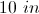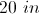Cannot be determined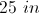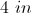Cannot be determined

Explanation:

Without more information we cannot determine the width of a rectangle based on its area alone.

If we know this is a square and therefore that all sides are equal, we could simply take the square root of 100, which is 10.

However, we do not know that all sides are equal. We need to know the length of the rectangle to solve this problem. Therefore the best we can do is see what the equation would look like if we knew the length.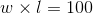or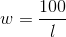where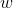is the width andis the length.

Note: the incorrect answer choices are all factors of 100 and possible answers, but we can't be sure which, if any, are the right one.

### Example Question #2 : How To Find The Length Of The Side Of A Rectangle

The area of a rectangle is 40; its width is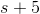. Which of the following expressions is equal to the length of the rectangle?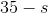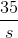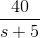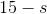Explanation:

Letbe the length. The area of the rectangle is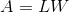. Replace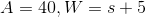and solve for: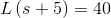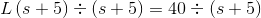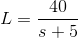### Example Question #3 : How To Find The Length Of The Side Of A Rectangle

The perimeter of a rectangle is 70; its width is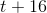. Which of the following expressions is equal to the length of the rectangle?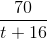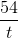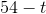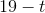Explanation:

Letbe the length. The perimeter of the rectangle is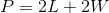.

Replace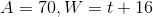and solve for: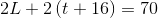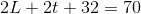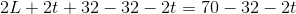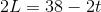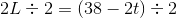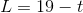### Example Question #1 : How To Find The Length Of The Side Of A Rectangle

A rectangle has perimeter 56. Its length is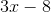; give its width in terms of.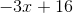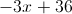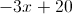Insufficient information is given to answer the question.Explanation:

Substitute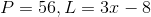in the formula for the perimeter of a rectangle: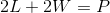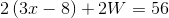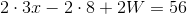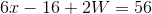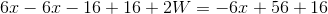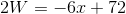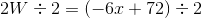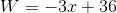### Example Question #5 : How To Find The Length Of The Side Of A Rectangle

You have a shed that you use for storing your gardening supplies and outdoor tools. If the shed has a footprint of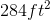and a width of 16 ft, what is the length of the shed? (assume the base is a rectangle)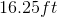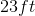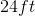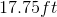Explanation:

You have a shed that you use for storing your gardening supplies and outdoor tools. If the shed has a footprint ofand a width of 16 ft, what is the length of the shed? (assume the base is a rectangle)

We are given a rectangle with a known area and width, and asked to find the length.

To do so, recall this formula: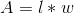We know A and w, so simply work backwards to solve for l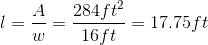So, our answer is 17.75 ft